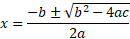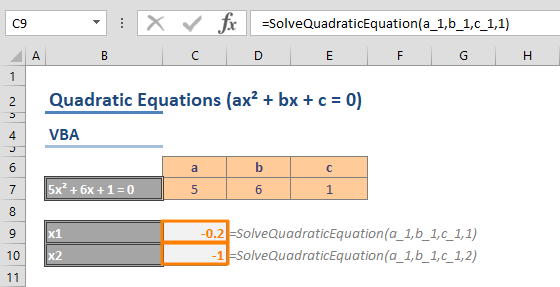This is the second part of the how to solve quadratic equations in Excel guide. Here, we are going to be focusing on how to do this manual and VBA method, whereas the first part shows how to do this using the Goal Seek feature. In this guide, we are going to show you a different approach to solving quadratic equations in Excel using formulas and VBA.

If you missed the first part:

A quadratic function is a type of equation that contains a squared variable. It is called quadratic because quad means square in Latin. The quadratic functions usually have a structure like ax² + bx + c = 0, where x represents an unknown variable, and a, b, and c represent known constants. Excel can help you easily solve these types of equations for x.

A quadratic function's variable can take 2 values, meaning that there can be 2 solutions. To find these values, you can use the quadratic formula:The plus/minus operator (±) means the formula should be executed twice. Once with plus (+):And once with minus (-):The a, b and c values are known numbers where a ≠ 0. Since we know the formula representation and the values, we can now create a formula for solving quadratic equations.

## The Standard Formula

For this first approach, you essentially need to replicate the quadratic formula in Excel. You can enter the known values (a, b and c) right away into the formula. However, this means that the formula will be static and you will need to change the values one-by-one again when you need to solve for another equation.

Alternatively, you can pull the input values from cells to place the constants a, b and c and use those references inside the formula. Changing the cell value is usually a lot easier than changing all values one-by-one, especially with complex formulas.

Let's assume that our constant values are in cells C7, D7 and E7. In this example, we also named these cells as a_1, b_1 and c_1 to make the formula easier to read. The formulas will be,

=(-b_1 + SQRT(POWER(b_1,2) - 4*a_1*c_1)) / (2*a_1)

=(-b_1 - SQRT(POWER(b_1,2) - 4*a_1*c_1)) / (2*a_1)

As you can see, one of the formulas uses plus and the other uses minus. This is how you can create a structure to solve the quadratic equations using traditional formulas. You can learn more about the POWER and SQRT functions in the respective pages.## Solving Quadratic Equations using VBA

The standard formula method is effective and dynamic. However, it may be hard to remember or use. While Excel doesn't have a function that allows solving quadratic equations with one click, you can create yourself one!

To start working with VBA, you need to enable the Visual Basic for Applications (VBA) window. When your workbook is open, press the Alt + F11 key combination. Once the VBA window is active, add a module. Modules are pages where you can write your VBA code.

Copy and paste the code below into the module you've just added:

Function SolveQuadraticEquation(a As Integer, b As Integer, c As Integer, result As Integer)
If result = 1 Then
SolveQuadraticEquation = (-b + Sqr(b * b - 4 * a * c)) / (2 * a)
ElseIf result = 2 Then
SolveQuadraticEquation = (-b - Sqr(b * b - 4 * a * c)) / (2 * a)
Else
SolveQuadraticEquation = "Invalid result value. It should be 1 or 2."
End If
End Function

This code creates a new function named SolveQuadraticEquation. This function has 4 arguments - 3 for known values, and 1 for selecting the plus/minus sign.After pasting the code, return to the Excel window and test your new function. First, enter the 3 known values, a, b and c. Set 1 or 2 to select between plus and minus respectively.